# Chern form

## Definition

### Setup

Let$M$ be a differential manifold,$E$ a complex vector bundle over$M$, and$\nabla$ a connection on$E$. Let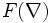$F(\nabla)$ denote the Riemann curvature tensor of$\nabla$, and$n$ denote the rank of$E$.

### Definition part

The Chern form of the pair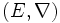$(E,\nabla)$, denoted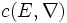$c(E,\nabla)$, is defined as:$c(E,\nabla) := det(1_E + \frac{i}{2\pi}(F(\nabla)))$$c$ is even-dimensional, viz it is a sum of elements from even-dimensional cocycles. The member from the$(2k)^{th}$ cochain group is denoted$c_k(E,\nabla)$. Special formulae exist for the first and last member: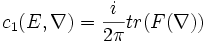$c_1(E,\nabla) = \frac{i}{2\pi} tr(F(\nabla))$

and: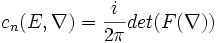$c_n(E,\nabla) = \frac{i}{2\pi} det(F(\nabla))$

The Chern-Weil theorem gives the basic facts about the Chern form that also establish its importance. In particular, it shows that the cohomology classes of the Chern forms are independent of the choice of connection.